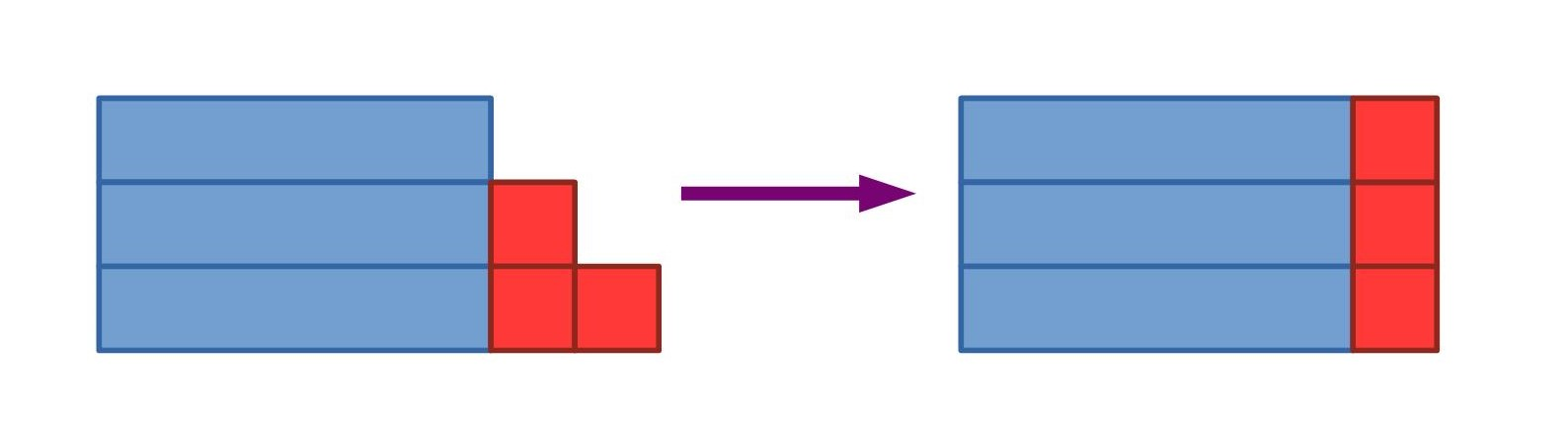#### You may also like### DOTS Division

Take any pair of two digit numbers x=ab and y=cd where, without loss of generality, ab > cd . Form two 4 digit numbers r=abcd and s=cdab and calculate: {r^2 - s^2} /{x^2 - y^2}.### Novemberish

a) A four digit number (in base 10) aabb is a perfect square. Discuss ways of systematically finding this number. (b) Prove that 11^{10}-1 is divisible by 100.### Latin Numbers

Can you create a Latin Square from multiples of a six digit number?

# Number Rules - OK

##### Age 14 to 16Challenge Level

Try some numbers first - are you convinced?

Can you see how the pictorial and algebraic methods below can be used to explain why the sum of three consecutive numbers is always a multiple of three?

Method 1:Method 2:

$$n+(n+1) + (n+2) = 3n + 3 = 3(n+1)$$

Try to use similar methods to explain the statements in the problem.